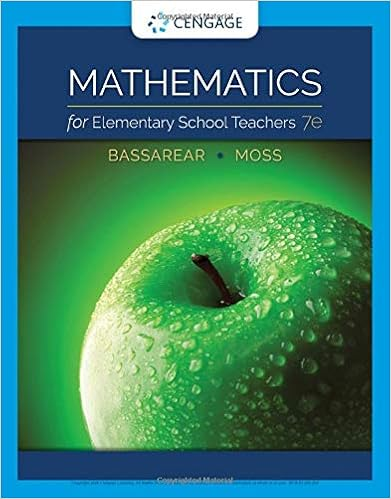Ask the students to do the construction on chart

• bert6761
• 88

This preview shows page 59 - 63 out of 88 pages.

We have textbook solutions for you!
The document you are viewing contains questions related to this textbook.The document you are viewing contains questions related to this textbook.
Chapter 4 / Exercise 38
Mathematics for Elementary School Teachers
BassarearExpert Verified
Ask the students to do the construction on chart paper. This can be done as a group activity. Ask students to construct a line segment AB of length 12 cm. Then, using a protractor, show them how to construct a perpendicular bisector on the board. A length of more than half the length of the line segment is taken and arcs are drawn above and below the line segment. The students can be asked to imagine that they are drawing a date tree above and below the line. The points where the arcs at the top and bottom intersect each other are joined by a line using a ruler. The halves of the line segment are measured and groups that get two exactly congruent halves are awarded points. This can be repeated at least five times and the group with the most points wins.
We have textbook solutions for you!
The document you are viewing contains questions related to this textbook.The document you are viewing contains questions related to this textbook.
Chapter 4 / Exercise 38
Mathematics for Elementary School Teachers
BassarearExpert Verified
iv 56 1 Construction of Line Segments Written Assignments Exercise 11b can to be done for homework in their notebooks. Evaluation A short assessment on construction can be given. Marks should be given for neat, accurate, and correctly labelled diagrams. After completing this chapter, students should be able to: identify different geometric instruments and their function: ruler, compasses, divider, protractor, and set-square, construct a line segment using a ruler and a pair of compasses, bisect line segments, and draw a perpendicular to a line.
v 57 1 12 Angles Specific Learning Objectives Types of angles Adding angles at a point Using a protractor to measure and construct angles Constructing an angle bisector Suggested Time Frame 4 to 5 periods Prior Knowledge and Revision Students know that angles and their different types. They also have knowledge of measuring angles, if not of constructing them. Real-life Application and Activities Starter Activity The teacher can give them a timed activity in which they are given three minutes to identify and note down all the angles as they can spot in the classroom. The teacher can first revise the following key angle facts, and then time them and see how many observations are made. Angles are formed by two rays. The two rays meet at one point which is called the vertex. An angle is denoted by the sign . When labelling an angle by three letters in upper case, the middle letter should denote the vertex. A protractor is used to measure an angle.
iv 58 1 Angles Example When a door opens, an angle is formed. The walls in a room meet at right angles. An angle is formed between the blades of a fan. The table legs are right angles to the floor. Summary of Key Facts An acute angle is less than 90°. A right angle is exactly 90°. An obtuse angle is more than 90° and less than 180°. A straight angle is equal to 180°. A reflex angle is more than 180° and less than 360°. Adjacent angles lie next to each other. Adjacent angles on a straight line are called supplementary angles.
•••